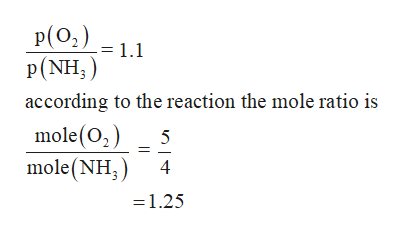# 4 NH3 (g) + 5 O2 (g) = 4 NO (g) + 6 H2O (g)A sample of NH3 is added to an initially evacuated 2.00 L flask at 125 C, until the pressure if 415 torr. O2 is then added to the flask until the total initial pressure ( before reaction) is 875 torr. Assuming the reaction goes to completion, find the partial pressure of all gases in the mixture, after the reaction is complete.

Question
27 views

4 NH3 (g) + 5 O2 (g) = 4 NO (g) + 6 H2O (g)
A sample of NH3 is added to an initially evacuated 2.00 L flask at 125 C, until the pressure if 415 torr. O2 is then added to the flask until the total initial pressure ( before reaction) is 875 torr. Assuming the reaction goes to completion, find the partial pressure of all gases in the mixture, after the reaction is complete.

check_circle

Step 1

Given that,

Partial pressure of NH3= 415torr

Partial pressure of O2 = (875-415) torr

= 460 torr

Step 2

The given reaction is

Step 3

As per the reaction

4 moles of NH3 reacts with 5 moles of O2

B...help_outlineImage TranscriptioncloseP(O2) = 1.1 P(NH) according to the reaction the mole ratio is mole(02) mole(NH 5 4 1.25 fullscreen

### Want to see the full answer?

See Solution

#### Want to see this answer and more?

Solutions are written by subject experts who are available 24/7. Questions are typically answered within 1 hour.*

See Solution
*Response times may vary by subject and question.
Tagged in

### Gas laws